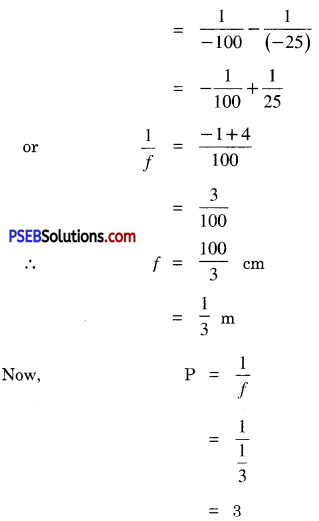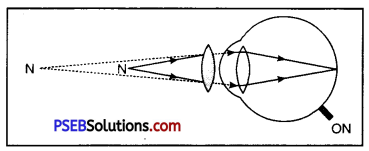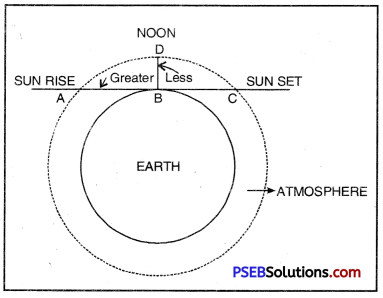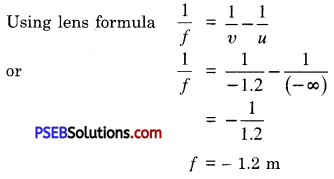# PSEB 10th Class Science Solutions Chapter 11 The Human Eye and The Colourful World

Punjab State Board PSEB 10th Class Science Book Solutions Chapter 11 The Human Eye and The Colourful World Textbook Exercise Questions and Answers.

## PSEB Solutions for Class 10 Science Chapter 11 The Human Eye and The Colourful World

PSEB 10th Class Science Guide The Human Eye and The Colourful World Textbook Questions and Answers

Question 1.
The human eye can focus object at different distances by adjusting the focal length of the eye lens. This is due to :
(a) presbyopia
(b) accommodation
(c) near-sightedness
(d) far-sightedness.
(b) accommodation.

Question 2.
The human eye forms the image of an object at its :
(a) cornea
(b) iris
(c) pupil
(d) retina.
(d) retina.

Question 3.
The least distance of distinct vision for a young adult with normal vision is about:
(a) 25 m
(b) 2.5 cm
(c) 25 cm
(d) 2.5 m.
(c) 25 cm.

Question 4.
The change in focal length of an eye lens is caused by the action of the :
(a) pupil
(b) retina
(c) ciliary muscles
(d) iris.
(c) ciliary muscles.Question 5.
A person needs a lens of power – 5.5 D for correcting his vision. For correcting his near vision, he needs a lens of power + 1.5 D. What is the focal length of lens required for correcting
(i) distant vision and
(i) For distant vision, f1 = $$\frac{1}{\mathrm{P}_{1}}$$
= $$\frac{1}{-5.5}$$
= – 0.182 m
⇒ f1 = – 18.2 cm

(ii) near vision?
For near vision, f2 = $$\frac{1}{\mathrm{P}_{2}}$$
= $$\frac{1}{1.5}$$
= + 0.667 m
f2 = + 66.7 cm

Question 6.
The far point of a myopic person is 80 cm in front of the eye. What is the nature and power of the lens required to correct the problem?
Here
Distance of far point u = – ∞
Focal length of the lens (v) = – 80 cm;

Using Less formula $$\frac{1}{f}=\frac{1}{v}-\frac{1}{u}$$
$$\frac{1}{(-80)}-\frac{1}{(-\infty)}$$ [∵$$\frac{1}{\propto}$$ = 0]
$$-\frac{1}{80}$$
Focal Length of the Lens (f) = – 80 cm = – 0.8 m
Negative sign (-) indicates that the nature of required lens is concave

Now,
P = $$\frac{1}{f}$$
= $$\frac{1}{-0.8}$$
P = – 1.25 D

Question 7.
Make a diagram to show how hypermetropia is corrected. The near point/ of hypermetropic eye is 1 m. What is the power of the lens required to correct this defect? Assume that the near point of normal eye is 25 cm.
u = – 25 cm,
v = – 1 m
= – 100 cm
Using lens formula $$\frac{1}{f}=\frac{1}{v}-\frac{1}{u}$$∴ Power = + 3 D
Convex (convergent) lens of power + 3 D

Question 8.
Why is a normal eye not able to see clearly the objects placed closer than 25 cm?
For seeing near objects, the ciliary muscles contract to make the lens thicker so as to reduce the focal length of eye lens, in order to form the image on the retina. Ciliary muscles cannot be contracted beyond certain limit and hence we cannot see clearly the objects lying closer than 25 cm, called the least distance of distinct vision.

Question 9.
What happens to the image distance in the eye when we increase the distance of an object from the eye?
For a fixed focal length of the lens, the distance of image from the lens (i.e., v) decreases as the distance of the object from eye lens (i.e., u) is increased.

Since v cannot be decreased (as distance between eye lens and retina is fixed), f is decreased by action of ciliary muscles so as to satisfy lens formula in accordance with increased value of u.Question 10.
Explain atmospheric refraction. Why do stars twinkle?
Twinkling of the star is due to refraction of light from stars through atmosphere. The stars emit their own light. The light coming from the stars on entering the earth’s atmosphere undergoes refraction continuously at each layer of atmosphere having different density, before it reaches the earth. The stars are very much distant objects and may be considered as point sources. The refractive index of the air changes from time to time due to change of density of air. Due to change of the optical density of earth’s atmosphere, the path of rays from stars continuously changes. The apparent positions of the stars continuously change due to change in refractive index of the atmosphere. Thus the stars appear to twinkle.

Question 11.
Why planets do not appear twinkling?
Since planets are quite close to the earth in comparison with the stars, they do not act as point sources but behave like extended sources. Planets may be considered as collection of a large number of point-sized sources of light. The total net deviation in amount of light entering our eyes from different point sources is zero. Therefore there is no net twinkling of planets.Question 12.
Why does the sun appear reddish in the morning (as well as in evening)?
Or
Why does rising star appears red in colour?
Earth is surrounded by envelope of gases called atmosphere. At the time of sunrise (or at sunset), light has to travel greater distance [AB at sunrise and BC at sunset] through the atmosphere to reach us than what it covers, when sun is over-head at noon [It has to travel only DB],

The wavelength of blue colour is about half that of red, blue light is scattered nearly 24 =16 times more than red colour of sunlight. As a result, the sun appears red at sunrise or at sunset due to negligible scattering of red colour of light.Question 13.
Why does the sky appears dark instead of blue to an astronaut?
The atmosphere exists only upto some height. At high altitudes, there is no atmosphere to scatter sun light. The sky, therefore, appears to be perfectly black to astronauts.

Science Guide for Class 10 PSEB The Human Eye and The Colourful World InText Questions and Answers

Question 1.
What is meant by power of accommodation of the eye?
Power of accomodation of eye. It is the ability of the eye lens to adjust its focal length to see various objects lying at different distances. The minimum distance upto which a normal eye can see clearly is called near point. For normal eye, near point is about 20 cm. The maximum distance upto which the eye can see clearly is called far point. The distance between near point and far point is also called accommodation.

Question 2.
A person with myopic eye cannot see objects beyond 1.2 m distinctly. What should be the type of the corrective lens used to restore proper vision?
Here u = – ∞; v = – 1.2 ; f =?Negative sign (-) shows that the corrective lens is concave lens.
But P = $$\frac{1}{f}$$
= $$-\frac{1}{1.2}$$ = – 0.825 D
The person should wear spects mounted with concave lens having of power – 0.825 D

Question 3.
What is the far point and near point of human eye with normal vision?